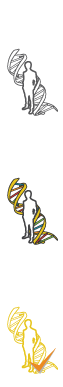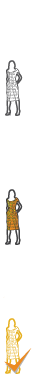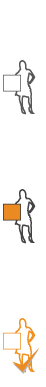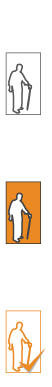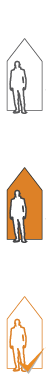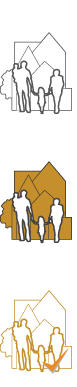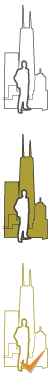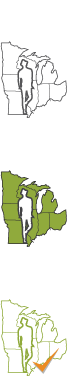#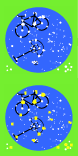2D Geometry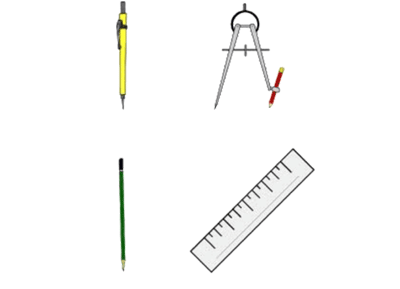To see the working of geometry is to see the design of nature. To understand the make-up of geometry, we must first know how it is constructed. Every shape you can think of is made from a series of points. Points make lines, lines make planes and planes make objects. Every object in the universe can be located by its points but we must first know where to begin.

Geometry is the mathematical study of shapes, figures and positions in space. Basic shapes are the line, the circle, the triangle, and the square. The point is the smallest unit of geometry. It takes three parts to make a line segment: a beginning, middle and end. A circle is a series of all points in a plane at a fixed distance (radius) from the center point. Planes have length (x) and height (y). Objects in space are things like points, lines, and angles. Perpendicular lines are lines or line segments that intersect or meet at 90 degrees. A ray is a line with one fixed point and one directional arrow.

Remember - plane geometry is two dimensional but we live in a three dimensional world!

## Activity 1 – Magic of the Point and LineGeometry orders our universe, but we first need to be able to recognize it. We measure geometry with a compass and a line. Place two points horizontal from each other and draw a line between them. Take your compass and draw two arcs with their centers on each of your two end points (be certain that the arc is larger than half the distance between the two points). The arcs will cross each other. Next, draw a line, it should be vertical, to connect the two points where the arcs meet. You now have divided your original line in half. You can use this method to not only divide a line into two but also to draw a perfect square or rectangle. Can you figure out how?## Activity 2 – Find the Origin and Divide the World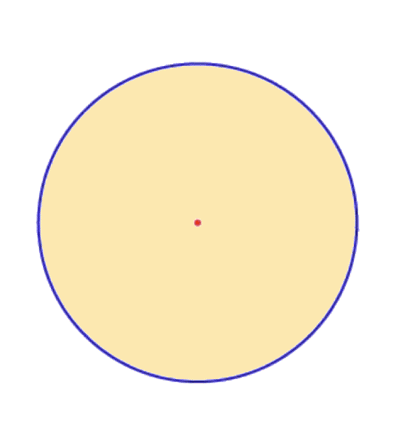You need a pencil, a straight edge, a compass and a hard flat surface. Begin by drawing a line, straight and simple. Pick a point on that line, anywhere. This is now your origin. Use your compass to draw a circle around your origin. Put the point of your compass on the origin and expand the compass outward. Keeping the point on the origin, rotate the compass 360 degrees. Next, draw two lines, one horizontal, one vertical, from your point of origin to the outside edge of the circle. You have just divided the circle into four quadrants. Finally, connect the outside points of your line to form a square around the circle and draw a diagonal line from the four corners to the opposite corner and then again to the opposite end of your original vertical line. You should see a square with a circle around it, a cross through the middle, with an X and a V in the center. From this diagram, you can find the angles to divide any circle into equal parts from one to ten.## Activity 3 – Design Your Own Geometry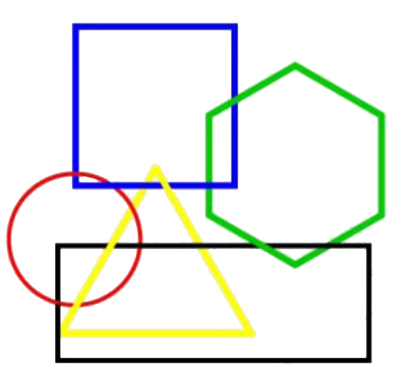Divide a piece of paper into four quadrants with a vertical line and a horizontal line. Place nine dots in three lines of three dots, in each quadrant; you will have 36 dots total, in a regular pattern. Next, draw your own polygons. Draw some with three sides, five sides or even ten sides. Draw some regular polygons (a closed shape with equal sides), irregular polygons (a closed shape with unequal sides), concave shapes (a dented shape) and convex shapes (a shape with a bulge). Then, label each shape with a number. Create a key along one side of your paper to test your friends. Test each other; see how many shapes they can name!## Activity 4 – Geometry of Constellations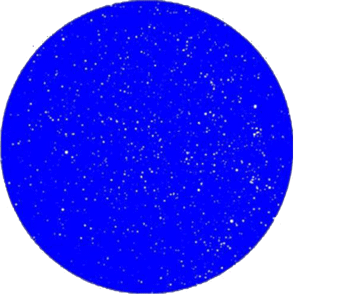Geometry orders our universe, but we must first be able to recognize it. We measure geometry with a compass and a line. People who came before us connected the stars above into geometric constellations. InvestigateYour Skyand click on the city where you live. Download a map of the night sky above your home. Be sure to un-check the Constellation Display option so you can create your own patterns. Print your overhead star map and use your pencil and a straight edge to connect the brightest stars. What sort of images do you see? An animal? Some sort of vehicle? A person? Write your name in the stars. Claim a piece of sky for yourself! Be sure to label your constellations so others can find the images you have discovered.## Activity 5 – Geometric Shapes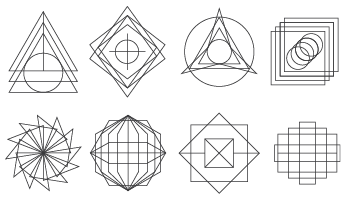Now that you can find shapes in your house, classroom, neighborhood and city, its time to experiment with more complex shapes. Try different polyhedrons, or shapes that are closed. Try overlapping them and shifting them. Nature at all scales is full of minute geometric relationships. If you explore the Pattern, Nature Patterns and Proportion Journeys you will find geometry in the yourself and everywhere in the world!

Geometry helps organize life!## Review

• The smallest geometric unit is:
• Perpendicular lines are lines that meet at a right angle (90 degrees).
• Lines have a beginning point, a middle point and an end point.
• Lines are always straight.
• Lines can never intersect.